medium post

## Sunday, October 22, 2017

### Optimizing deeper networks with KFAC in PyTorch.

Medium post.

(I'm getting too much comment spam on Blogger, so I'll probably use medium/something else from now on, and just link here)

## Tuesday, May 12, 2015

### ICLR 2015

Some ICLR posters that caught my eye:

[larger image]
Very simple to implement idea that gives impressive results. They force two groups of units to be uncorrelated by penalizing their cross covariance. When the first group is also forced to model classes, the second group automatically models the "style". The problem if separating out "style" has been studied for a while, see  Tenenbaum's "Separating style and content with bilinear models" and reverse citations, but this implementation is the simplest I've seen and could be implemented as a simple modification of batch-SGD.

[larger image]

Similar to Yann LeCun's "Siamese Network" but potentially makes learning problem easier by looking at 2 pairs of examples at each step -- two examples of same class + two examples of different class. The loss models these pair distances -- first distance must be smaller.

[larger image]

Apparently Adobe has collected photographs of text manually tagged with font identity, which authors promise to release at http://www.atlaswang.com/deepfont.html . Biased here because I've looked at fonts before (ie, see notMNIST dataset).

[larger image]

Similar to James Martens Optimizing Neural Networks with Kronecker-factored Approximate Curvature. Both papers make use of that fact that if you want to white gradient in some layer, it's equivalent (but cheaper) to whiten activations and backprop values instead. Martens inverts activation covariance matrix directly, Povey has an iterative scheme to approximate it with something easy to invert.

[larger image]

With Async SGD workers end up having stale values that deviate from what the rest of the workers look at. Here they derive some guidance on how far each worker is allowed to deviate.

[larger image]

They get better results by doing SGD where examples are sampled using non-uniform strategy, such as sampling in proportion to gradient magnitude. This is empirical confirmation of results from Stochastic Gradient Methods workshop last year, with people showing optimal SGD sampling strategies related to "take high gradient magnitude steps first"

These are essentially separable ConvNets, where instead of learning regular filters and then trying to approximate them with low rank expansion, they learn rank-1 filters right away. Such decrease in parameters while keeping same performance and architecture is dramatic and surprising.

[larger image]

The most intriguing paper of the conference IMHO. It's an alternative to backprop for training networks. The basic idea is this -- your neural network is a function

$$f_\text{dataset}(\text{parameters})=\text{labels}$$

In ideal world, you could learn your labels by inverting f, then your estimate is
$$\text{new_params}=f^{-1}(\text{labels})$$

That's too hard, so you approximate $f$ with a quadratic function with unit Hessian $g$ and invert that and let

$$\text{newparams}=g^{-1}(\text{labels})$$

Since original approximation was bad, new estimate will be off, so you repeat the step above. This gives you standard gradient descent.

An alternative approach is to try to learn $g^{-1}$ from data directly. In particular, notice that in a standard autoencoder trained to do $f(g(x))=x$, $g$ an be viewed as an inverse of $f$. So in this paper, they treat neural network as a composition of functions corresponding to layers and try to invert each one using approach above.[larger image]

Biased because I've played with Theano, but apparently someone got Theano running well enough to train ImageNet in similar speed to Caffe (30% slower on 1 GPU from some beer conversations)

If you have 10k classes, you'll need to take dot product of your last layer activations with 10k rows of the last parameter matrix. Instead, you could use WTA hashing to find a few rows likely to produce dot product with your activation vector, and only look at those.

Nvidia demoing their 4-GPU rig.

[larger image]

Use some off-the-shelf software to decompose 4d convolutional tensor (width-height-input depth-output depth) into faster to compute Tensor product. However, if Flattened convolutional networks work for other domains, this seems like an overkill.

[larger image]

They took network trained on ImageNet and sent regions of the image that activated by particular filters to labelers, to try to figure out what various neurons respond to. A surprisingly high number of neurons learned semantically meaningful concepts.

This was further reinforced by demo by Jason Yosinski showing visualization of various filters in ImageNet network. You could see emergence of "Face" and "Wrinkle" detectors, even though no such class is in ImageNet

## Wednesday, March 05, 2014

Last week I attended Stochastic Gradient Methods workshop held at UCLA's IPAM . Surprisingly, there's still quite a bit of activity and unsolved questions around what is essentially, minimizing a quadratic function.

In 2009 Strohmer and Vershinin rediscovered an algorithm used for solving linear systems of equations from 1970 -- Kaczmarz method, and showed that this algorithm is a form of Stochastic Gradient. This view of SGD motivates a biased sampling strategy which gives faster convergence rate than regular Stochastic Gradient. This spurred a flurry of activity, motivating results in at least 5 different lectures.

In 2010, Nesterov showed that Randomized Coordinate Descent has a faster convergence rate than SGD, and in 2013 Singer showed a way to accelerate it to quadratic convergence. In 2013 Richtarik gave an alternative algorithm to get the same convergence rate, but also comes up with better step sizes that rely on sparsity pattern of the problem.

Summaries of talks I attended with links to slides are below:

### Ben Recht

Gave an overview of Hogwild and Jellyfish methods. Hogwild has been covered a few times before at NIPS, but here's an overview slide

Jellyfish (described in their Large Scale Matrix completion paper) chooses sampling order in a way to minimize lock contention.

Also talked about their work on explaining the gap between performance of SGD sampling with replacement vs. without replacement. Empirically, without replacement works better (see Section 5 of "Beneath the valley" paper) yet until recently tools were missing to explain it. They are able to prove faster rates of no-replacement sampling for Kaczmarz algorithm by relying on "Noncommutative arithmetic-geometric mean inequality."

#### Resources:

• Slides Recht - we should all run hogwild!.pdf
• Beneath the valley of the noncommutative arithmetic-geometric mean inequality: conjectures, case-studies, and consequences. http://arxiv.org/abs/1202.4184
• Parallel Stochastic Gradient Algorithms for Large-Scale Matrix Completion. Recht and Re. 2011.
• HOGWILD!: A Lock-Free Approach to Parallelizing Stochastic Gradient Descent. Niu, Recht, Re, and Wright. 2011.

### Yoram Singer

Talked about accelerating coordinate descent with momentum-like approach, dubbed Generalized Accelerated Gradient Descent. Nesterov's accelerated gradient method has quadratic convergence with linear dependence on condition number of the loss
$$O\left(\frac{L}{k^2}\right)$$

Parallel coordinate descent depends on average of per-coordinate Lipschitz constants, which can be much better for badly conditioned loss:
$$O\left(\frac{\bar{L_i}}{k}\right)$$

The methods proposed has quadratic convergence of accelerated gradient, meanwhile retaining dependence on average curvature, rather than the worst
$$O\left(\frac{\bar{L_i}}{k^2}\right)$$

### Dimitri Bertsekas

In-depth tutorial "Incremental Gradient, Subgradient, and Proximal Methods for Convex Optimization: A Unified Framework"

One slide that stuck out is the one-dimensional illustration of why SGD works.In the farout region, all gradients are pointing in the same direction, so taking gradient step with respect to a single component function works just as well as looking at the full sum.

This also serves as the motivation for "heavy ball" method (Polyak, 1964). When you are in farout region, you want to accelerate, while in confusion region, you want to decelerate, you can accomplish this by modifying gradient update formula as follows

$$x_{x+1} = x_k-\alpha_k \nabla f_{i_k}(x_k)+\beta_k(x_k-x_{k-1})$$

This is similar in spirit to "Accelerated Stochastic Approximation" of Kesten (1958) which grows the step size if the difference between successive $x$'s is the same sign, and shrinks if there are many sign changes.

Schmidt said Stochastic Averaged Gradient works better than Kesten's approach in a multi-dimensional setting.

### Peter Richtarik

Gave overview of his "Accelerated, Parallel and Proximal Coordinate Descent". It gives a technical improvement over his previous work "Distributed coordinate descent method for learning with big data" (http://arxiv.org/abs/1310.2059) which seems to have the meat of the contributions.

Here's a slide from his talk comparing various methods."Prox" column means the algorithm can take proximal steps, i.e., can be used with constraints and not-nice regularizers. "Accel" or Accelerated is whether the method is enjoys $O(1/k^2)$ convergence rate where $k$ is the iteration counter. "General f" means it applies for convex problems rather than quadratic. "Block" is whether method can update some of the coordinates at a time rather than all coordinates.

Setting of the problem is summarized in slide belowYou are optimizing a sum of losses $f_e$, and not all losses depend on all examples. You want to update your sets of coordinates in blocks, in parallel. Sets of variables involved for each $f_e$ determine how well you can parallelize the problem. In half-a-dozen papers on his website he develops framework dubbed Expected Separable Overapproximation (ESO) to analyze such problems.

One outcome of ESO approach is a formula that incorporates sparsity of the problem into calculation of step size. See Table 3 of his approx paper (http://arxiv.org/pdf/1312.5799v1.pdf)

$$v_i = \sum_{j=1}^m (1+\frac{(\omega_j-1)(\tau-1)}{\max(1,n-1)}A_{ji}^2$$

This is formula for step size for coordinate $i$ in randomized coordinate descent, computed as a sum over examples $j$. Quantity $\omega_j$, is the number of components of vector that example $x_j$ depends on, $n$ is dimensionality, and $\tau$ is the number of coordinates are updated in parallel. $A$ is the matrix of quadratic minimization problem, replaced with matrix of per-coordinate Lipschitz constant for general convex problems.

### Rachel Ward and Deanna Needell

Gave background on their paper "Stochastic Gradient Descent and the Randomized Kaczmarz Algorithm". The setting:Further in presentation, developed importance sampling for SGD. Traditionally, SGD picks random component of the sum above, and the number of steps required to reach given accuracy is proportional to the worst condition number (Lipshitz constant) over per-example losses.

Derived following formula for the number of steps needed to reach given accuracy $\epsilon$ with uniform sampling

$$k \propto \log \epsilon (\sup_i \frac{L_i}{\mu} + \epsilon^{-1} \frac{\sigma^2}{\mu^2})$$

For quadratics, the first term is close to largest condition number out of all component functions $f_i$, except you are normalizing by global smallest eigenvalue $\mu$, rather than per-component smallest eigenvalue $\mu_i$. The second term is "normalized consistency" - expected squared norm of the squared gradient divided by smallest eigenvalue squared.

Instead of uniform sampling, we can sample examples in linear proportion to Lipshitz constant for gradient of loss on that example. This cuts down the number of steps needed to average Lipschitz constant, normalized by strong convexity parameter $mu$, rather than largest Lipschitz constant. Since Lipschitz constant is the upper bound on the largest eigenvalue of the Hessian, this means number of steps grows in proportion to average condition number rather than the largest condition number.

The term involving Lipschitz constant now drops from max to average. In other words we get this:
$$\sup_i \frac{L_i}{\mu} \to \frac{\bar{L}}{\mu}$$

The second (consistency) term can instead potentially get larger, we get
$$\frac{\sigma^2}{\mu^2}\to \frac{\bar{L}\sigma^2}{\inf_i L_i \mu^2}$$

The best trade off depends on details of function -- badly conditioned, but accurate gradients -- sample proportionally to Lipshitz. Well conditioned and noisy gradients, do closer to uniform. She shows that if we sample halways between uniform and Lipshitz, so called "partially biased sampling", both terms are guaranteed to be smaller than for uniform sampling.

Yuriy Nesterov obtained similar bounds for sampling strategy and convergence in his "Efficiency of coordinate descent methods on huge-scale optimization problems". Key difference is that he samples which coordinate to update at each step, instead of sampling examples. Optimal sampling strategy comes down to picking coordinates in linear proportion to their Lipschitz constant, and the convergence rate also drops to the average of per-coordinate Lipschitz constants rather than the worst Lipschtitz constant. Roughly speaking, number of steps till convergence goes down to average eigenvalue of Hessian rather than worst eigenvalue.

Deanna Needell gave background on the Kaczmarz Algorithm which gives alternative way to motivate importance sampling results. In particular, first few slides illustrate why the order matters. She also gives analytic expression to find the best next point to sample for the quadratic case. This requires $O(\text{# of examples})$ search at each iteration. She then shows approximation approach based on dimensionality reduction that takes $O(1)$ time per step.

Ben Recht's made a similar point on impact of choosing better ordering in his presentation### Stephen Wright

Started with a nomenclature discussion on how "Stochastic Gradient Descent" methods don't qualify as gradient descent, because SGD steps can be in ascent directions for the global cost function. Instead, they should be referred to as "Stochastic Gradient" methods. Every speaker afterwards corrected themselves on the usage.

Gave overview of parallel Kaczmarz method and then extended analysis to get convergence rate for parallel Kaczmars with "Inconsistent Read" allowed -- situation where the parameter vector gets modified while it's being read.

### Yann LeCun

Gave background on convolutional neural networks and showed demo of online learning using ImageNet. Basically it was network running network pre-trained on ImageNet, and using nearest neighbor in the embedding induced by activations of the last layer.Impressively, it seems to do a good job learning to recognize from just a single example.

Also talked about connections between neural network learning and random matrix theory. You can see the connection if you rewrite activations of ReLU neural network as follows

$$\sum_P C(x) \prod_{(i,j) \in P} W_{i,j}$$

The sum is over all paths through active nodes from input layer to output node. Coefficients $C_x$ depend on input data. This is a polynomial with degree equal to the number of layers, and there are results from random matrix theory says that if coefficients $C(x)$ are Gaussian distributed, then local minima are close together in energy, so essentially, finding local minimum is as good as finding global minimum.

### Francis Bach

Presented results on convergence rates of SGD and how they are affected by lack of strong convexity.

### Jorge Nocedal

Talked about adaptation of quasi-Newton method to stochastic setting. Convergence of SGD depends on square of condition number, meanwhile Newton's method is independent of condition number, at the cost of step size that that costs $O(\text{dimensions}^2)$

The compromise he proposes is to do BFGS-like method where
1. You use exact Hessian information to compute product of Hessian and step direction
2. You only do it once every 20 iterations

This makes the cost of l-BFGS-like update similar to SGD update.

### Asuman Ozdaglar

Introduced a way to extend ADMM to graph-structured problems without having to choose the order of updates. The setting of problem is summarized belowAs you may recall, ADMM works by decoupling components of the loss by having each loss operate on their own copy of the parameters. You alternate between each function minimizing itself locally with their own copy of parameters, and setting values of parameters locally from the functions that have already been minimized.

This steps can be implemented as message passing on a factor graph -- factors here are components of the cost function, whereas nodes are variables that the cost function depends on. Each component function depends on a subset of variable, and each variable is involved ina  subset of component functions.

Implementation of ADMM is similar to Divide and Concur, where a readable overview is given in Yedidia's Message-Passing paper.One inconvenience of this approach is that it requires establishing an arbitrary order of message updates.

Ozdaglar's idea is to introduce the symmetry by adding extra variables, one for each direction of the constraint variable, and adding an extra constraint that forces them to agree. The update is done in parallel, like parallel BP, followed by an extra step that synchronizes the extra constraint variables.

### John Duchi

John Ducchi gave a white-board talk on convergence of 0-th order optimization. Happily, the convergence is only a factor of sqrt(dimensions) worse than standard SGD.

Started with succinct derivation of non-asymptotic error of proximal average algorithm, which looks a lot like averaged SGD, after $k$ steps, in terms of errors of gradients. The actual formula has no O-terms and proof is found in notes, but roughly it looks like this

$$E(\text{error}) <= O(\frac{1}{\sqrt{k}})+\frac{1}{k}\sum_{i=1}^{k} E[\|\epsilon_i\|]$$

Error here is in terms of value of the function, which is what we care about in applications (as opposed to distance from true parameter vector). As $k$ increases, the second term vanishes and you get the regular $1/\sqrt{k}$ convergence. If you don't care about constraints, "prox" step can be replaced by an SGD step.

### Mark Schmidt

Gave an overview of their Stochastic Averaged Gradient algorithm. Full details and many extensions are in their hefty 45 page arxiv paper.

Their motivation is a method to combine fast initial convergence for stochastic method, and fast late-stage convergence of full-gradient methods, while keeping cheap iteration cost of stochastic gradient.

Stochastic Averaged Gradient reaches this goal with a simple modification of stochastic gradient. The idea is that at each gradient step, in addition to the gradient computed for the current data point, you also add up all the gradients computed on previous datapoints. Those gradients may be out of date, but for strongly convex loss with convex component functions, this staleness doesn't hurt.

Schmidt et al advocated sampling datapoints with high curvature more often based on the argument that such gradient might be changing faster, and needs to be evaluated more often. However, the formal justification of this intuition is not avaiable, and instead they fall back on the same analysis as Kaczmarz importance sampling described earlier.

One difference of weighted sampling from standard SGD setting is that examples can be sampled more often without needing to correct for this bias because the weight of each gradient is $1/n$ in SAG regardless of how many times the function is sampled. However, bias correction will come up as an issue in any large scale adaptation of SAG when you can't store all gradients in memory.

### Lin Xiao

Gave an overview of stochastic variance reduction gradient methods. The idea of variance reduction is to periodically evaluate full gradient, and then use it to adjust future gradient steps. If we evaluated full gradient at previous point $\tilde{x}$, formula for gradient update becomes as follows

$$x_{k+1}=x_k - \nu (\nabla f_{i_k} - \nabla f_{i_k}(\tilde{x})+\nabla F(\tilde{x}))$$

Here $\nabla{F(\tilde{x})}$ is the full gradient evaluated at some previous point $\tilde{x}$, $\nabla{f_{i_k}(x_k)}$ is the gradient evaluated at loss for current example $x_k$.

The idea of variance reduction is illustrated below.On the left you see what would happen if you applied gradient reduction formula with $\nabla{F(\tilde{x})}$ computed at each step. That reduces to regular gradient descent. If instead we evaluate full gradient once every $k$ iterations, the correction will be based on stale value of gradient and not quite correct, however the mean error is zero so it gives an unbiased estimate of the correction term.

Then they introduce a weighted sampling strategy, where datapoints are sampled proportionally to the condition number of individual loss functions. When number of iterations is much larger than number of examples, weighted sampling strategy drops convergence to $O(C_{\text{avg}}$ steps as opposed to $O(C_\max)$ steps for uniform sampling, C_{\text{avg}} is the average condition number over all component loss functions.

### James Spall

Gave results on Stochastic Approximation methods. Aproximation can be seen as minimization of distance between solution and ideal solution, so SA methods come down to some form of stochastic optimization. The difference is that the setting is more general - non-convexity, can't compute gradients, possibly discrete problem.

Standard approach to derivative free methods is Finite Difference Stochastic Approximation (FDSA) where to numerically compute gradient, you do $2p$ function evaluations where $p$ is dimensionality.

The idea of Simultaneous Perturbation Stochastic Approximation method (SPSA) is to evaluate gradient along randomly chosen directions, and take step in that directions with step-length proportional to gradient in that direction. This requires two function evaluations instead of $2 p$ for FDSA, and works just as well.

Two summary slides from the SPSA talk:Here was a graph of numerical simulation of SPSA vs standard approachHe gave a more in-depth overview of the methods in 2012 NIPS talk. It's available as youtube video, but here are screenshots of some intro slides.

Simple SPSA is essentially a first order method, and has the same problems as other first order methods:

• sensitivity to upscaling of units of $\theta$
• slow convergence in the final phase

To address these, he introduces Adaptive Stochastic Approximation by the Simultaneous Perturbation Method (ASP) which goes further by numerically estimating the Hessian in addition to the gradient.

The approach to approximating Hessian is similar in spirit to SPSA -- compute gradient in two random directions, and estimate the Hessian numerically from that (formula 2.2 in "Adaptive Stochastic Approximation by the Simultaneous Perturbation Method"). This requires 4 function evaluations.

This estimate is noisy, so use momentum to smooth Hessian estimates.

More recent work (Spall 2009) gives an improved formula for estimating the Hessian numerically using what he calls "feedback term".

Adaptive SPSA methods store Hessian approximation explicitly, like BFGS, so they aren't directly applicable to deep neural nets.

## Friday, December 06, 2013

### Deep Learning Internship at Google, Summer 2014

We have a couple of internship openings for someone to train deep neural nets find extract interesting things in StreetView imagery. The ideal person would come and push the envelope of what's possible with large amount of training data (billions of labeled image examples for some tasks), and large amount of computation power data (essentially unlimited when you parallelize). If you are interested, first apply through links below, and after passing the screen, contact me directly at yaroslavvb@gmail.com (feel free to share this post) For PhDs: https://www.google.com/about/jobs/search/#!t=jo&jid=3481001& For Masters: https://www.google.com/about/jobs/search/#!t=jo&jid=3480001&﻿

## Tuesday, October 30, 2012

### Summer Intern opening

We are looking for a summer intern to apply Deep Learning techniques to the problem of reading text in the wild. More details here

## Tuesday, May 01, 2012

### The Average Font

I came across this post post where the author created a font by averaging together all fonts on his machine. I thought it would be cool to do the same for all fonts on the internet -- here's the average of about 375k distinct fonts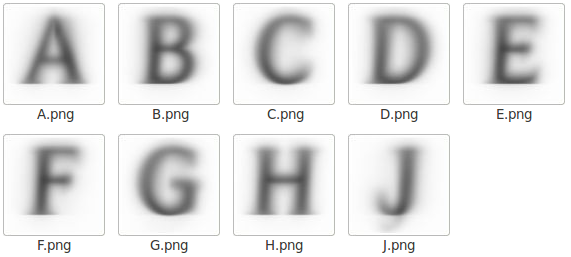It's interesting that shapes are clearly seen even though fonts on the web are quite noisy, here's a random sample of things that make up the A above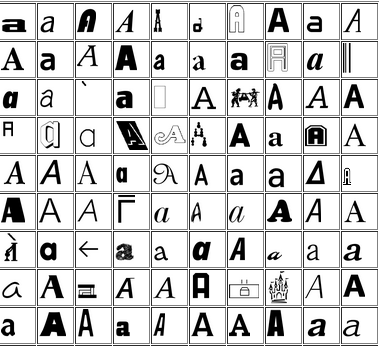## Monday, November 21, 2011

### Interesting papers coming up at NIPS'11

There's a number of accepted papers whose camera-ready versions have been posted already. Here are the ones I found interesting. I'll give further update on these after the conference. Additionally, here's a non-NIPS preprint I came across that summarizes belief propagation and related algorithms. In particular, it turns out that state of the art algorithm for sphere packing, Divide and Concur is closely related to belief propagation.

## Sunday, November 13, 2011

### Shapecatcher

Here's a cool tool I stumbled across reading John Cook's blog -- Shape Catcher looks up Unicode value from a drawing of a character.

Apparently it uses Shape Context features.

This motivated me to put together another dataset, unlike notMNIST this focuses on the tail end of Unicode, this is 370k bitmaps representing 29k Unicode values, grouped by Unicode

## Wednesday, November 09, 2011

This is a dataset of scans of 1000 public domain books that was released to the public at ICDAR 2007. At the time there was no public serving infrastructure, so few people actually got the 120GB dataset. It has since been hosted on Google Cloud Storage and made available for public download
• [filename] goes from Volume_0000.zip to Volume_0999.zip

import os
for i in range(1000):
if count>1: break
fname = 'Volume_%04d.zip'%(i)
f = 'curl -L http://commondatastorage.googleapis.com/books/icdar2007/%s -o %s'%(fname,fname)
os.system(f)

The goal of this dataset is to facilitate research into image post-processing and accurate OCR for scanned books.

## Sunday, November 06, 2011

### b-matching as improvement of kNN

Below is an illustration of b-matching from (Huang,Jebara AISTATS 2007) paper. You start with a weighted graph and the goal is to connect each v to k u's to minimize total edge cost. If v's represent labelled datapoints, u's unlabeled and weights correspond to distances, this works as a robust version of kNN classifier (k=2 in the picture) because it prevents any datapoint from exhibiting too much influence. They show that this restriction significantly improves robustness to changes in distribution between training and test set. See Figure 7 in that paper for an example with MNIST digits. This is just one of a series of intriguing papers on matchings that came out of Tony Jebara's lab, there's a nice overview on his page that ties them together.

## Tuesday, October 25, 2011

My group has intern openings for winter and summer. Winter may be too late (but if you really want winter, ping me and I'll find out feasibility). We use OCR for Google Books, frames from YouTube videos, spam images, unreadable PDFs encountered by the crawler, images from Google's StreetView cameras, Android and few other areas. Recognizing individual character candidates is a key step in OCR system. One that machines are not very good at. Even with 0 context, humans are better. This shall not stand!

For example, when I showed the picture below to my Taiwanese coworker he immediately said that these were multiple instance of Chinese "one".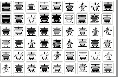Here are 4 of those images close-up. Classical OCR approaches, have trouble with these characters.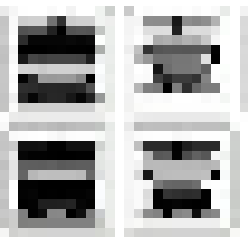This is a common problem for high-noise domain like camera pictures and digital text rasterized at low resolution. Some results suggest that techniques from Machine Vision can help.

For low-noise domains like Google Books and broken PDF indexing, shortcomings of traditional OCR systems are due to
1) Large number of classes (100k letters in Unicode 6.0)
2) Non-trivial variation within classes
Example of "non-trivial variation"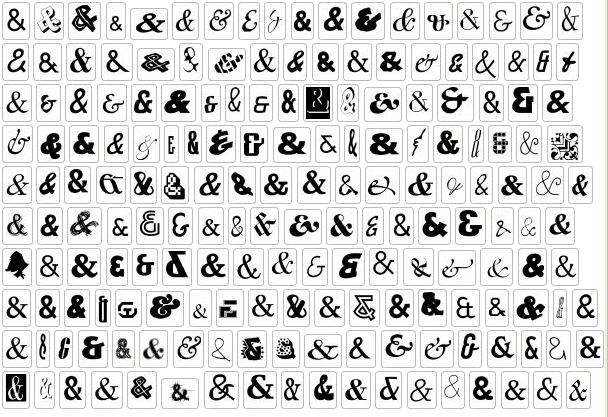I found over 100k distinct instances of digital letter 'A' from just one day's crawl worth of documents from the web. Some more examples are here

Chances are that the ideas for human-level classifier are out there. They just haven't been implemented and tested in realistic conditions. We need someone with ML/Vision background to come to Google and implement a great character classifier.

You'd have a large impact if your ideas become part of Tesseract. Through books alone, your code will be run on books from 42 libraries. And since Tesseract is open-source, you'd be contributing to the main OCR effort in the open-source community.

You will get a ton of data, resources and smart people around you. It's a very low bureocracy place. You could run Matlab code on 10k cores if you really wanted, and I know someone who has launched 200k core jobs for a personal project. The infrastructure also makes things easier. Google's MapReduce can sort a petabyte of data (10 trillion strings) with 8000 machines in just 30 mins. Some of the work in our team used features coming from distributed deep belief infrastructure.

In order to get an internship position, you must pass general technical screen that I have no control of. If you are interested in more details, you could contact me directly.

## Saturday, September 24, 2011

### Don't test for exact equality of floating point numbers

A discussion came up on Guido von Rossum's Google Plus post. It comes down to the fact that 2.1 is not exactly represented as a floating point number. Internally it's 2.0999999999999996, and this causes unexpected behavior.

These kinds of issues often come up. The confusion is caused by treating floating point numbers as exact numbers, and expecting calculations with them to produce results meaningful down to the last bit.

People at Wolfram Research has spent a lot of effort trying to get arithmetic right, and here's what a principle kernel developer, Daniel Lichtblau, has to say about the issue of making floating point calculations deterministic:

It is not in any way "solvable", at least not by means accessible to us (which in some sense defines it as "not a problem"). Depends too much on alignment-handling vagaries of MKL libraries, ordering of operations in BLAS, and usage, or not, of extended precision registers. See also IEEE 754: a careful reading may shed light on how different results for the same computation can arise in compliant hardware/software, even on the same machine

In addition to ambiguity in IEEE 754, which gives slight differences between different floating point libraries, there's an issue of the same library giving slightly different results on reruns with same inputs and and same machine, because of run-time optimization by the processor

The solution that Wolfram Inc came up with is to treat the last 7 bits of IEEE doubles as unknown. When testing for equality, those bits are ignored. When printing a number, it chooses representation that gives a nice printout. For instance, Print[2.0999999999999996] will display 2.1

So here's the rule of thumb for IEEE 754 floating point numbers:

When checking for equality of floating point doubles, ignore the last 7 bits of the mantissa.

## Thursday, September 08, 2011

### notMNIST dataset

I've taken some publicly available fonts and extracted glyphs from them to make a dataset similar to MNIST. There are 10 classes, with letters A-J taken from different fonts. Here are some examples of letter "A"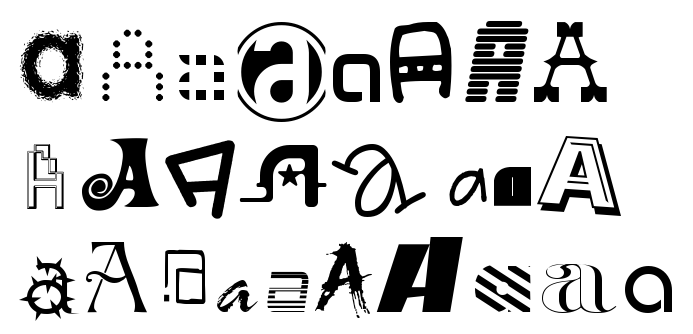Judging by the examples, one would expect this to be a harder task than MNIST. This seems to be the case -- logistic regression on top of stacked auto-encoder with fine-tuning gets about 89% accuracy whereas same approach gives got 98% on MNIST. Dataset consists of small hand-cleaned part, about 19k instances, and large uncleaned dataset, 500k instances. Two parts have approximately 0.5% and 6.5% label error rate. I got this by looking through glyphs and counting how often my guess of the letter didn't match it's unicode value in the font file. Matlab version of the dataset (.mat file) can be accessed as follows:
load('notMNIST_small.mat')
for i=1:5
figure('Name',num2str(labels(i))),imshow(images(:,:,i)/255)
end

Zipped version is just a set of png images grouped by class. You can turn zipped version of dataset into Matlab version as follows
tar -xzf notMNIST_large.tar.gz
python matlab_convert.py notMNIST_large notMNIST_large.mat

Approaching 0.5% error rate on notMNIST_small would be very impressive. If you run your algorithm on this dataset, please let me know your results.

## Tuesday, August 16, 2011

### Making self-contained Unix programs with CDE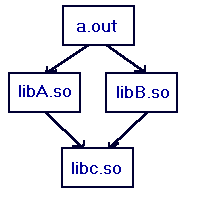In the old days you could statically link your program and run it on another Unix station without worrying about dependencies. Unfortunately static linking no longer works, so you need to make sure that your target platform has the right libraries.

For instance, in order to get Matlab compiled code running on a server, you have to copy over libraries and set environment variables as specified in Matlab's guide, followed by some investigative work to take care of dependencies and version conflicts not covered by Mathworks documentation.

Another example is when if you want to create a self-contained Scipy/Python environment that you could just copy over and use without needing to run configure and make.

For both cases Philip Guo's CDE tool comes helpful. It analyzes your program to find dependencies and makes a package with all the dependencies included. Then it runs the program through a kind of lightweight virtualization, intercepting program calls to trick it into thinking it's running in the original environment.

For instance, here's how you could make a self-contained environment for Python and Scipy:

cd /temp
git clone git://github.com/pgbovine/CDE.git
cd CDE
make
cd ..
CDE/cde python -c "from scipy import *; print zeros(15)"
tar -cf package.tar cde-package


This makes a 26 MB file that includes that all files that were read during execution of that command.
Now you can move it to another machine that doesn't have scipy, prepare the environment and try it out as follows
tar -xf package.tar
cd cde-package/cde-root/temp
../../cde-exec python -c "from scipy import *; print ones(15)"


This will work even if machine doesn't have Python installed, or has the wrong version of shared libraries installed, because cde-exec is reproducing the environment of the original machine. It works for anything that doesn't need files other than the ones accessed during package creation.

For instance, you could start an interactive Python session and run some SciPy examples like this

cde cde-package/cde-root
../cde-exec python
>>> from scipy import *
>>> ....


## Wednesday, July 13, 2011

Google+ seems to have a fair number of Machine Learning people, I was able to track down 50 people I've met at conferences by starting at Andrew McCallum's circles. If you add me on Google Circles I'll assume you came from this blog and add you to my "Machine Learning" circle

## Saturday, June 25, 2011

### Embracing non-determinismComputers are supposed to be deterministic. This is often the case for single processor machines. However, as you scale up, guaranteeing determinism becomes increasingly expensive.

Even on single processor machines you are facing non-determinism on semi-regular basis. Here are some examples

• Bugs + poor OS memory control that allows programs to read uninitialized memory. A recent example for me was when I used FontForge to process a large set of files, and had it crash on a different set every time I ran it.
• /dev/random gives "truly random" numbers, however, even if the program uses regular rand() seeded by time, it's essentially non-deterministic since so many factors affect the time of seed()
• Floating point operations on modern architecture are non-deterministic because of run-time optimization -- depending on the pattern of memory allocation, processor will rearrange the order of your arithmetic operations, producing slightly different results on reruns
• Soft-errors in circuits due to radiation, about one error per day, according to stats here
• Some CPU units are bad and produce incorrect results. I don't know exact stats on this, but the guy who did the Petasort experiment told me that it was one of the issues they had to deal with.
.

Those modes of failure may be rare on one computer, but once you scale your computation up to many cores, it becomes a fact of life.

In a distributed application like sorting a petabyte of data, you should expect about 1 hardrive failure, 100 irrecoverable disk read errors, and a couple of undetected memory errors if you use error correcting RAM (more if you use regular RAM).

You can make your computation deterministic by adding checksums at each stage and recomputing the parts that fail. However, this is expensive, and becomes disporportionately more expensive as you scale up. When the Czajkowski et al sorted 100 trillion records deterministically, most of the time was spent on error recovery.

I've been running an aggregation that is modest in comparison, going over over about 100 TB of data and getting slightly different results each time. Theoretically I could add recovery stages that get rid of non-determinism, but at a cost that is not justified in my case.

Large scale analysis systems like Dremel are configured to produce approximate results by default, otherwise, the long tail of packet arrival times means that you'll spent most of the time waiting for a few "stragglers"

Given the increasing cost of determinism it makes sense to think of it in terms of cost-benefit analysis and trade off the benefit of reproducible results against the cost of 100% error recovery.

A related idea come up in Vikash Mansinghka presentation at NIPS 2010 workshop on monte carlo methods: Real world decision problems can be formulated as inference and solved with MCMC sampling, so it doesn't make sense to spend so much effort ensuring determinism only to destroy it with /dev/random -- you could achieve greater efficiency by using analogue circuits to start with. His company, Navia, is working in this direction.

There's some more discussion on determinism on Daniel Lemire's blog

## Wednesday, June 22, 2011

### Machine Learning opportunities at Google

Google is hiring and there are lots of opportunities to do Machine Learning-related work here. Kevin Murphy is applying Bayesian methods to video recommendation, Andrew Ng is working on a neural network that can run on millions of cores, and that's just the tip of the iceberg that I've discovered working here for last 3 months.

There is machine learning work in both "researcher" and "engineer" positions, and the focus on applied research makes the distinction somewhat blurry.

## Saturday, April 30, 2011

### Neural Networks making a come-back?

Five years ago I ran some queries on Google Scholar to see trends on the number of papers that mention particular phrase. The number of hits for each year was divided by the number of hits for "machine learning". Back then it looked like NN's started gaining in popularity with invention of back-propagation in 1980's, peaked in 1993 and went downhill from there.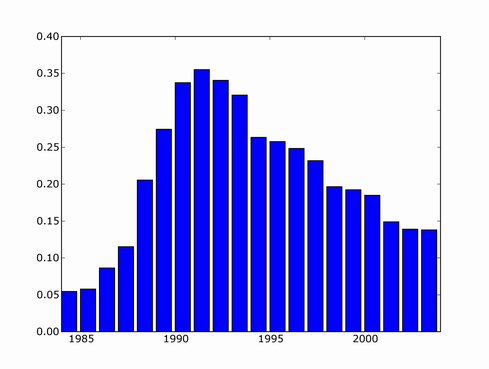Since then, there's been several major NN developments, involving deep learning and probabilistically founded versions so I decided to update the trend. I couldn't find a copy of scholar scraper script anymore, luckily Konstantin Tretjakov has maintained a working version and reran the query for me.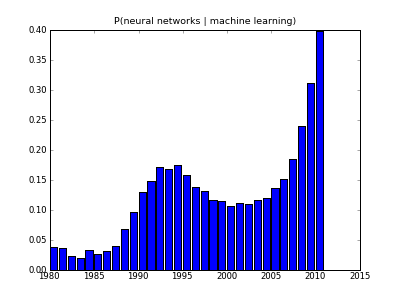It looks like downward trend in 2000's was misleading because not all papers from that period have made it into index yet, and the actual recent trend is exponential growth!

One example of this "third wave" of Neural Network research is unsupervised feature learning. Here's what you get if you train a sparse auto-encoder on some natural scene images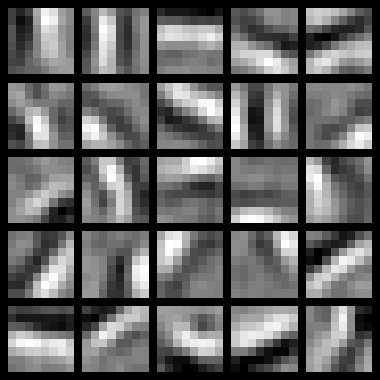What you get is pretty much a set of Gabor filters, but the cool thing is that you get them from your neural network rather than image processing expert

## Friday, April 29, 2011

### Another ML blog

I just noticed that Justin Domke has a blog --

He's one of the strongest researchers in the field of graphical models. I first came across his dissertation when looking for a way to improve loopy-Belief Propagation based training. His thesis gives one such idea -- instead of maximizing the fit of an intractable model, and using BP as intermediate step, maximize the fit of BP marginals directly. This makes sense since approximate (BP-based) marginals are what you ultimately use.

If you run BP for k steps, then likelihood of the BP-approximated model is tractable to minimize -- calculation of gradient is very similar to k steps of loopy BP. I derived formulas for gradient of "BP-approximated likelihood" in equations 17-21 in this note. It looks a bit complicated in my notation, but derivation is basically an application of chain rule to BP-marginal, which is a composition of k-steps of BP update formula.

## Sunday, March 13, 2011

I've accepted an offer from Google and will be joining their Tesseract team next week.

I first got interested in OCR when I faced a project at my previous job involving OCR of outdoor scenes and found it to be a very complex task, yet highly rewarding because it's easy to make incremental progress and see your learners working.

Current state-of-the-art OCR tools are not at human level of reading, partially because humans are able to take context into account. For instance, sentence below is unreadable if you don't assume English language.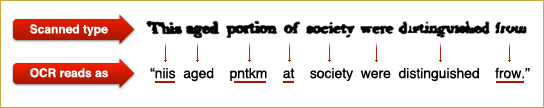Also, I'm excited to work on an open-source project. I'd like to live in a world where the best technologies are open-source and free to everyone.

## Saturday, March 05, 2011

### Linear Programming for Maximum Independent Set

Maximum independent set, or "maximum stable" set is one of classical NP-complete problems described in Richard Karp's 1972 paper "Reducibility Among Combinatorial Problems". Other NP-complete problems often have a simple reduction to it, for instance, p.3 of Tony Jebara's "MAP Estimation, Message Passing, and Perfect Graphs" shows how MAP inference in an arbitrary MRF reduces to Maximum Weight Independent Set.

The problem asks to find the largest set of vertices in a graph, such that no two vertices are adjacent.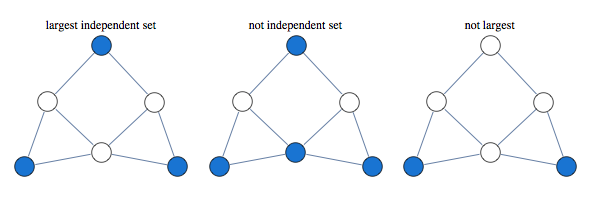Finding largest independent set is equivalent to finding largest clique in graph's complement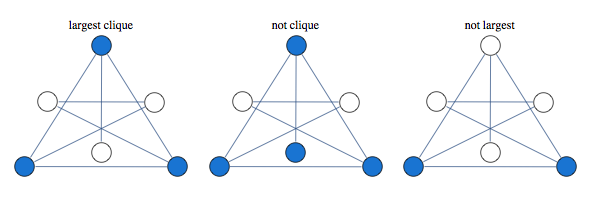A classical approach to solving this kind of problem is based on linear relaxation.

Take a variable for every vertex of the graph, let it be 1 if vertex is occupied and 0 if it is not occupied. We encode independence constraint by requiring that adjacent variables add up to 1. So we can consider the following graph and corresponding integer programming formulation: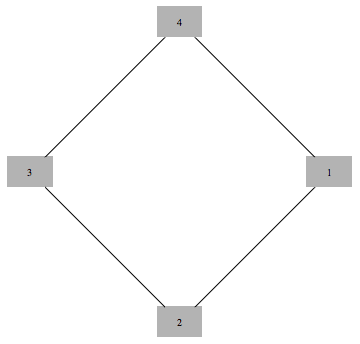$$\max x_1 + x_2 + x_3 + x_4$$

such that
$$\begin{array}{c} x_1+x_2 \le 1 \\\\ x_2+x_3 \le 1 \\\\ x_3+x_4 \le 1 \\\\ x_4+x_1 \le 1 \\\\ x_i\ge 0 \ \ \forall i \\\\ x_i \in \text{Integers}\ \forall i \end{array}$$

Solving this integer linear integer program is equivalent to the original problem of maximum independent set, with 1 value indicating that node is in the set. To get a tractable LP programme we drop the last constraint.

If we solve LP without integer constraints and get integer valued result, the result is guaranteed to be correct. In this case we say that LP is tight, or LP is integral.

Curiously, solution of this kind of LP is guaranteed to take only values 0,1 and $\frac{1}{2}$. This is known as "half-integrality". Integer valued variables are guaranteed to be correct, and we need to use some other method to determine assignments to half-integer variables.

For instance below is a graph for which first three variables get 1/2 in the solution of the LP, while the rest of the vertices get integral values which are guaranteed to be correct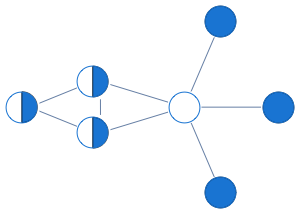Here's what this LP approach gives us for a sampling of graphs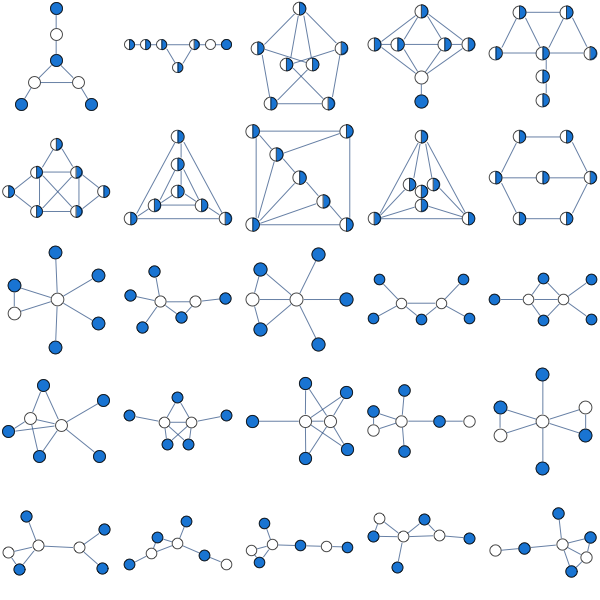A natural question to ask is when this approach works. Its guaranteed to work for bipartite graphs, but it also seems to work for many non-bipartite graphs. None of the graphs above are bipartite.

Since independent set only allows one vertex per clique, we can improve our LP by saying that sum of variables over each clique has to below 1.

Consider the following graph that our original LP has a problem with: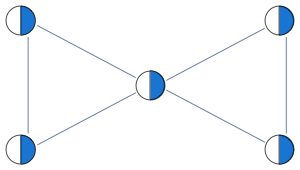Instead of having edge constraints, we formulate our LP with clique constraints as follows:

$$x_1+x_2+x_3\le 1$$
$$x_3+x_4+x_5\le 1$$

With these constraints, LP solver is able to find the integral solution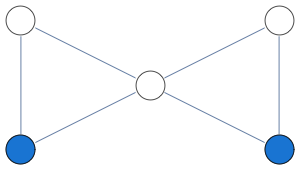In this formulation, solution of LP is no longer half-integral. For the graph below we get a mixture of 1/3 and 2/3 in the solution.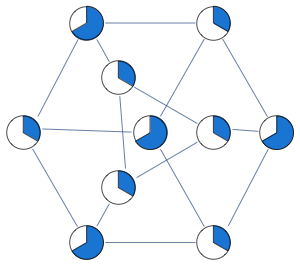Optimum of this LP is known as the "fractional chromatic number" and it is an upper bound both on the largest clique size and the largest independent set size. For the graph above, largest clique and independent set have sizes 9/3 and 12/3, whereas fractional chromatic number is 14/3.

This relaxation is tighter than previous, here are some graphs that previous LP relaxation had a problem with.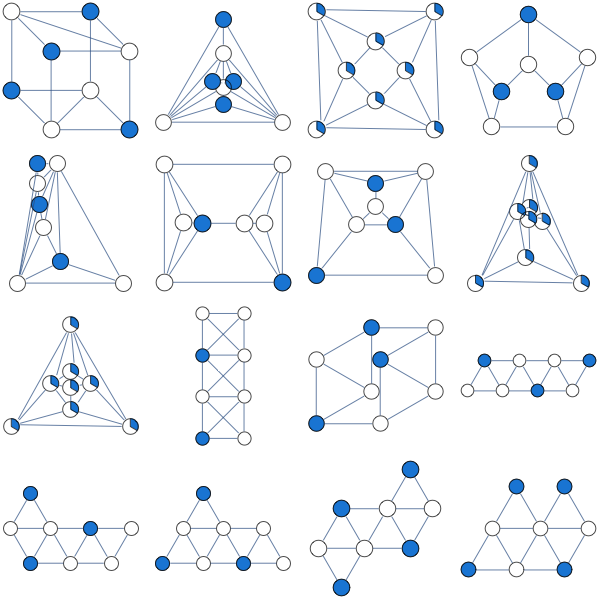This LP is guaranteed to work for perfect graphs which is a larger class of graphs than bipartite graphs. Out of 853 connected graphs over 7 vertices, 44 are bipartite while 724 are perfect.

You can improve this LP further by adding more constraints. A form of LP constraints that generalizes previous two approaches is

$$\sum_{i\in C} x_i\le \alpha(C)$$

Where $C$ is some subgraph and $\alpha(C)$ is its independence number.

You extend previous relaxation by considering subgraphs other than cliques.
Lovasz describes the following constraint classes (p.13 of "Semidefinite programs and combinatorial optimization")

1. Odd hole constraints
2. Odd anti-hole constraints
3. Alpha-critical graph constraints.

Note that adding constraints for all maximal cliques can produce a LP with exponential number of constraints.

Notebook
Packages